## TOONIFY

 Applies a cartoon-like effect to an image.

 USAGE: toonify [-b blur] [-t threshold] [-e edge] [-g gain] [-l lothresh] [-h hithresh] [-q quantize] [-s smoothing] [-B brightness] [-C contrast] [-S saturation] infile outfile USAGE: toonify [-help] -b ... blur ......... blur sigma for selective-blur; integer>=0; default=4 -t ... threshold .... threshold for selective-blur; 0<=integer<=100; default=10 -e ... edge ......... edge method; choices are: none, sobel, DoG, LoG and canny; ..................... default=sobel -g ... gain ......... gain for edge strength; integer>=0; default is automatically ..................... computed for different edge methods; not used for edge=canny -l ... lothresh ..... low threshold for canny edge method; integer>=0; default=5 -h ... hithresh ..... high threshold for canny edge method; integer>=0; default=20 -q ... quantize ..... quantization number of color levels; 0<=integer<=255; zero means ..................... no quantization; default=8 -s ... smoothing .... smoothing of quantization boundaries; float>=0; default=1 -B ... brightness ... brightness change; integer; default=0 -C ... contrast ..... contrast change; integer; default=0 -S ... saturation ... saturation increase; 0<=integer<=49; default=0 PURPOSE: To apply a cartoon-like effect to an image. DESCRIPTION: TOONIFY applies a cartoon-like effect to an image. Edges and color quantization are optional. There are several choices for the edge method. ARGUMENTS: -b blur ... BLUR sigma for selective-blur. Values are integers>=0. The default=4. -t threshold ... THRESHOLD for selective-blur. Values are 0<=integers<=100. The default=10. -e edge ... EDGE method. The choices are: none (n), sobel (s), DoG (d) (for difference of gaussians), LoG (l) (for log of gaussian) and canny (c). The default=sobel. -g gain ... GAIN for edge strength. Values are integers>=0. The default is automatically computed for different edge methods. For DoG it is 20. For LoG it is 15. For sobel it is 4. This option is not used for canny. -l lothresh ... LOTHRESH is the low threshold for the canny edge method. Values are integers>=0. The default=5. -h hithresh ... HITHRESH is the high threshold for the canny edge method. Values are integers>=0. The default=20. -q quantize ... QUANTIZE is the quantization number of color levels. Values are 0<=integers<=255. A value of zero means no quantization. The default=8. -s smoothing ... SMOOTHING of the quantization color boundaries. Values are floats>=0. The default=1. -B brightness ... BRIGHTNESS change. Value are integers. The default=0. -C contrast ... CONTRAST change. Values are integers. The default=0. -S saturation ... SATURATION increase. Values are 0<=integer<=49. The default=0. REFERENCES: http://www.cs.cornell.edu/courses/cs4670/2010fa/projects/final/results/group_of_pw272_zkd2/ComputerVisionFinalReport/ComputerVisionFinalWriteup.pdf https://stacks.stanford.edu/file/druid:yt916dh6570/Dade_Toonify.pdf CAVEAT: No guarantee that this script will work on all platforms, nor that trapping of inconsistent parameters is complete and foolproof. Use At Your Own Risk.

### EXAMPLES

 Example 1 -- Variation in Blur Original (source)Arguments: -b 1 -t 10 -e none -q 0 Arguments: -b 4 -t 10 -e none -q 0 Arguments: -b 16 -t 10 -e none -q 0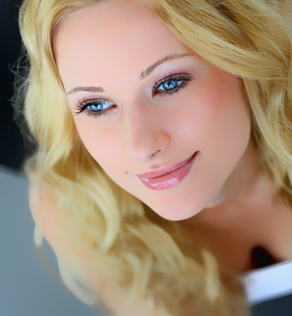Example 2 -- Variation in Threshold Original (source)Arguments: -b 4 -t 5 -e none -q 0 Arguments: -b 4 -t 10 -e none -q 0 Arguments: -b 4 -t 20 -e none -q 0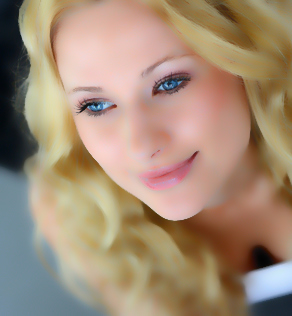Example 3 -- Variation in Edge Original (source)Arguments: -b 4 -t 10 -e DoG -q 0 Arguments: -b 4 -t 10 -e LoG -q 0Arguments: -b 4 -t 10 -e sobel -q 0 Arguments: -b 4 -t 10 -e canny -q 0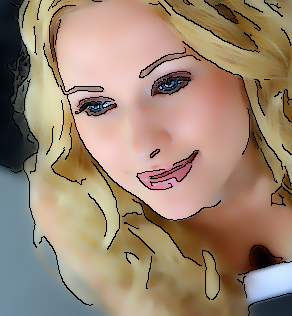Example 4 -- Variation in Edge with Quantization Original (source)Arguments: -b 4 -t 10 -e DoG -q 8 -s 2 Arguments: -b 4 -t 10 -e LoG -q 8 -s 2Arguments: -b 4 -t 10 -e sobel -q 8 -s 2 Arguments: -b 4 -t 10 -e canny -q 8 -s 2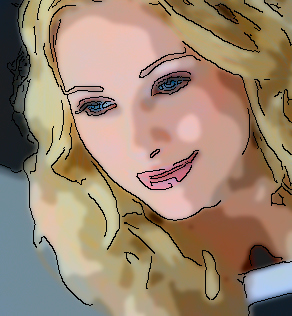Example 5 -- Variation in Edge with Quantization Original (source)Arguments: -b 4 -t 10 -e DoG -q 8 -s 1 -B 20 -S 10 Arguments: -b 4 -t 10 -e LoG -q 8 -s 1 -B 20 -S 10Arguments: -b 4 -t 10 -e sobel -q 8 -s 1 -B 20 -S 10 Arguments: -b 4 -t 10 -e canny -q 8 -s 1 -B 20 -S 10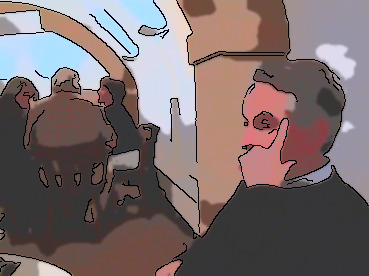Example 6 Original Arguments: -b 4 -t 10 -e sobel -q 8 -s 2Example 7 Original (source) Arguments: -b 4 -t 10 -e sobel -q 8 -s 1What the script does is as follows: Converts the input to LAB colorspace Applies selective-blur to the luminance channel Optionally clones the smoothed luminance channel and extracts edges Optionally color quantizes the smoothed luminance channel Replaces the old luminance channel with the smoothed one, deletes the old one, combines channels and converts to sRGB Optionally applies the edges to the image This is equivalent to the following IM commands convert -quiet "\$infile" \$tmpA if [ "\$saturation" = "0" ]; then satproc="" else loval=\$saturation hival=\$((100-saturation)) satproc="-level \${loval}x\${hival}%" fi if [ "\$brightness" = "0" -a "\$contrast" = "0" ]; then bcproc="" else bcproc="-brightness-contrast \${brightness},\${contrast}" fi if [ "\$edge" = "dog" ]; then [ "\$gain" = "" ] && gain=20 edgeproc="-define convolve:scale=!\$gain -morphology convolve DoG:0,0,1 -negate" elif [ "\$edge" = "log" ]; then [ "\$gain" = "" ] && gain=15 edgeproc="-define convolve:scale=!\$gain -morphology convolve LoG:0,1 -negate" elif [ "\$edge" = "sobel" ]; then [ "\$gain" = "" ] && gain=4 edgeproc="-define convolve:scale=!\$gain -define morphology:compose=Lighten \ -morphology Convolve Sobel:> -negate" elif [ "\$edge" = "canny" ]; then edgeproc="-canny 0x1+\${lothresh}+\${hithresh}% -negate" fi if [ "\$smoothing" = "0" ]; then smoothproc="" else smoothproc="-blur 0x\$smoothing" fi convert -respect-parenthesis \$tmpA -colorspace LAB -separate +channel \ \( -clone 0 -selective-blur 0x\${blur}+\${threshold}% \$bcproc \) \ \( -clone 3 \$edgeproc \) \ \( -clone 3 +dither -colors \$quantize \$smoothproc \) -swap 3,5 +delete \ \( -clone 2 -clone 1 \$satproc -clone 3 -reverse -set colorspace LAB -combine -colorspace sRGB \) \ -delete 0-3 -compose multiply -composite \ "\$outfile"